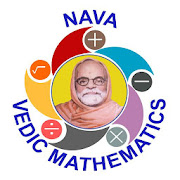# VEDIC MATHEMATICS LEVEL1Everyone
Nava Vedic Mathematics Level 1: This App introduces Vedic Mathematics in simple way with step by step explanation using two formats:

1. PDF Format – Text format
2. Classroom video – Audio and video format

The content of Nava Vedic Mathematics App Level 1 are:

INTRODUCTION TO VEDIC MATHEMATICS - Ancient India, Vedic Mathematics, Importance of Vedic Mathematics, Abacus and Vedic Mathematics, Sutras and Upa-Sutras

INTRODUCTION TO NUMBERS - Introduction, Classification, Basic Operators

LEARN TABLES IN SIMPLE WAY - Grid Table Method, Hand Method, Multiplication Table Trick For 7 And 8, Multiplication Table Trick For 2-Digit Numbers

INTERESTING CONCEPTS OF VEDIC MATHEMATICS - Introduction, Base ,Parama Mitra - Multiplication table using Paramamitra, Paramamitra for 9; Sum Root / Digital Root, Casting Out 9’s, Answer Verification – Addition, Subtraction, Multiplication, Division

DIVISIBILITY RULES - Introduction, Divisibility Rules for 1, 2, 3, 4, 5, 6, 8, 9, 10; Divisibility Rules for Prime Numbers - 7, 11, 13, 17 and 19; Divisibility Rules for Composite Numbers - 12, 14, 15, 16, 18,20, 21, 22, 24 and 25;

ADDITION - Introduction, Ekadhikena Purvena Sutra (2, 3 and 4 digits with Carryover); Vilokanam Sutra (2, 3 and 4 digits with Carryover); Nikhilam Sutra (2, 3 and 4 Digits)

SUBTRACTION- Introduction ,Nikhilam Navatashcaramam Dashatah Sutra (2, 3 and 4 Digits);EkadhikenaPurvena Sutra and Param Mitra (2, 3 and 4 Digits);EkanyunenaPurvena and Param Mitra (2, 3 and 4 Digtis);

MULTILPICATION - Introduction, Multiplying With 11 (2 digits with and without Carryover, 3 digits with and without Carryover); Multiplying with 12 (2 digits with and without Carryover, 3 digits with and without Carryover); Multiplying with 5 (2, 3 digits);Multiplying with 25 (2, 3 digits); Multiplying with 125 (2, 3 Digits); Multiplying With 9’s - Multiplying With 9 (2, 3 Digits), Multiplying With 99 (2, 3 Digits), Multiplying With 999 (2, 3 Digits)

DIVISION - Introduction, Dividing Number By 5 (2, 3 Digits); Dividing Number By 14 (2, 3 Digits); Dividing Number By 16 (2, 3 Digits)
Collapse

Review Policy

## What's New

Nava Vedic Mathematics Level 1: This App introduces Vedic Mathematics in simple way with step by step explanation using two formats:

1. PDF Format – Text format
2. Classroom video – Audio and video format
Collapse

Updated
April 22, 2019
Size
6.0M
Installs
100+
Current Version
9.2
Requires Android
4.0.3 and up
Content Rating
Everyone
Permissions
Offered By
Nava Vision
Developer
159/4, 2nd Floor, Sumathi Vital Nilaya, Vignana Nagar Main Road, Above Tasty Bite bakery, Vignana Nagar, Bangalore-75